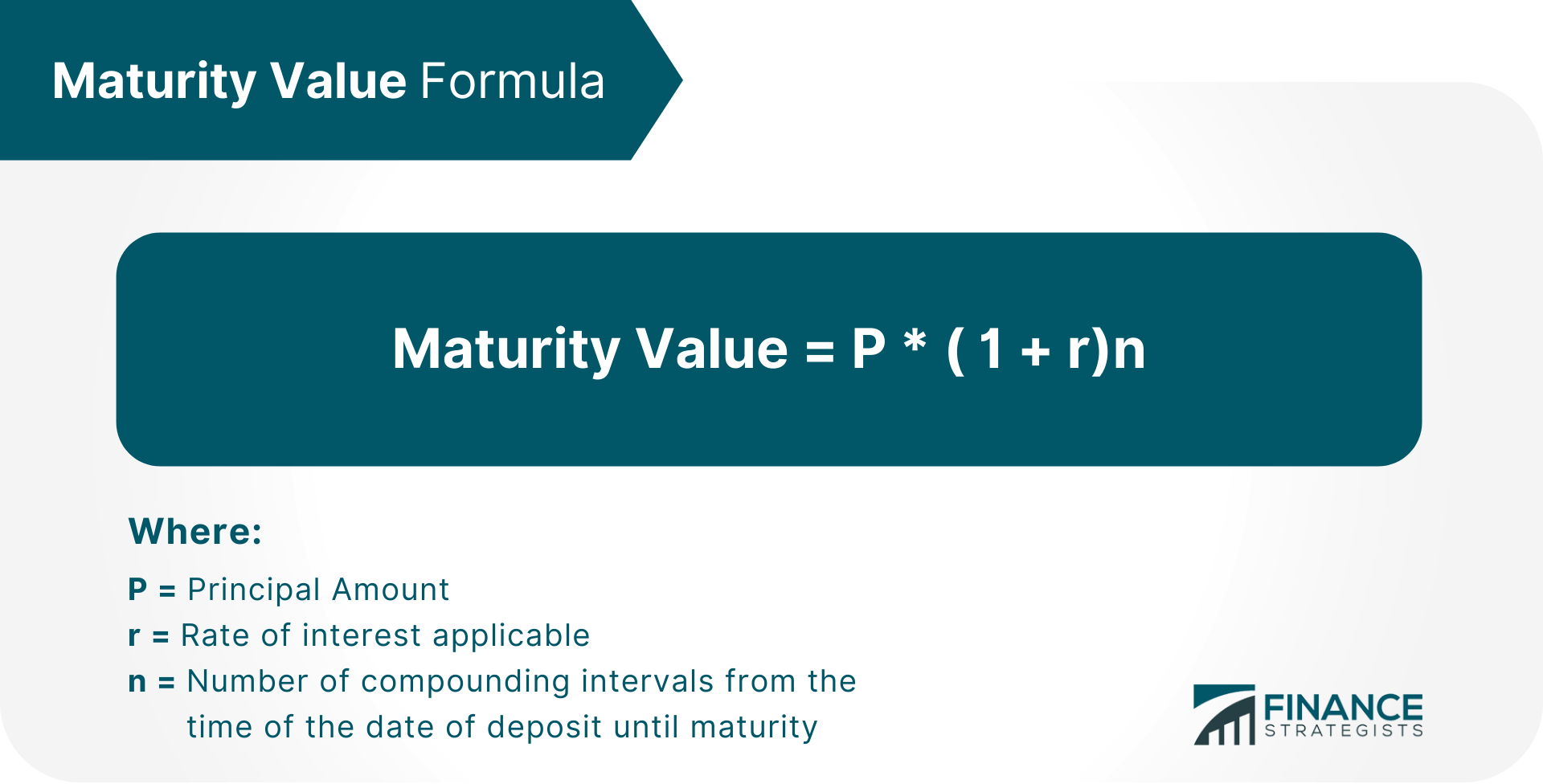# Maturity Value### Written byTrue Tamplin, BSc, CEPF® | Reviewed by Editorial Team

Updated on December 29, 2022

Maturity Value is the estimated future benefit of the investment at its scheduled date of maturity.

It is most often used to describe bank accounts, certificates of deposit, and other similar investments.

In simple terms, maturity value can be viewed as an "estimated future benefit."

## Why Does Maturity Value Matter?

Maturity values matter because they can impact your financial planning by clearly showing how much money you will have available at a given time in the future.

Such calculations can help you see whether or not you will have enough money to cover surprise emergencies, retirement income needs, and other financial goals.

## How Do I Calculate Maturity Value?

To calculate maturity value, you first need to know your investment's terms (e.g., the amount of money invested, interest rates, and due date).## Types of Maturity Values

There are two types of maturity values: "full" and "partial".

### Full

This is the most common type of maturity value. It assumes that all principal and interest earned through the end of the term will be reinvested into an account earning a similar rate.

### Partial

A partial maturity value only takes into account the amount of money invested, not any earnings on the principal (e.g., if the principal grows because of compound interest).

## When Should You Consider Using a Maturity Value?

The best way to determine when you should consider using a maturity value is to assess your financial planning situation.

If you are unsure about what long-term goals you should be saving for, maturity value calculations can help.

If you are comfortable making assumptions about the future value of your money, then you can use a maturity value to estimate how much money will be available in the future.

If not, then you may want to avoid using a maturity value.

## Factors that Affect the Growth of Maturity Value

When planning for the future, it is important to understand that there are a variety of factors that can impact how quickly a maturity value grows.

These include:

1. What kind of interest rates you're earning on your investment
2. How often the interest is compounded
3. How much time has passed since the deposit was made
4. What type of financial product you are using (i.e., what type of bank account or CD you have)
5. What the actual length of the term is, if it's shorter/longer than the original anticipated length
6. What fees are being deducted from your interest payments
7. What tax implications are involved (e.g., capital gains taxes on maturity value versus earned income tax on/after maturity)
8. What provisions are in place, if any, for a penalty-free early withdrawal or transfer of funds from this investment

All of these factors can impact how quickly your maturity value will grow and thus how long it will be until you have access to those funds.

Even with this knowledge, it is still beneficial to use a maturity value as one of many tools in your financial planning.

## Drawbacks to Using a Maturity Value

The downside of using maturity value, is that it does not consider the possibility of an early withdrawal, penalty-free.

Maturity values do not account for the possibility of changes to your estimated future benefit.

For example, if you are relying on your maturity value to cover an emergency expense and that amount is incorrect, then it may not be enough money to purchase the same goods/services at that time.

## Key Takeaways

Maturity values allow you to estimate the future value of money and thus help you better plan for your future financial needs.

Maturity values matter because they can impact your financial planning by clearly showing how much money you will have available at a given time in the future.

A full maturity value can be viewed as an "estimated future benefit".

There are a variety of factors that can impact how quickly a maturity value grows.

It is beneficial to use a maturity value as one of many tools in your financial planning, but it's important to understand not to make assumptions about the future value of your money.

## Maturity Value FAQs

### What is a Maturity Value?

Maturity value is the sum of future cash flows for investment.

### Why Does it Matter?

Maturity values matter because they can impact your financial planning by clearly showing how much money you will have available at a given time in the future.

### What are the Types of Maturity Values?

There are two types of maturity values: 'full' and 'partial'. A full maturity It assumes that all principal and interest earned through the end of the term will be reinvested into an account earning a similar rate. A partial maturity value only takes into account the amount of money invested, not any earnings on the principal.

### What is an Example of How to Use Maturity Value in My Situation?

Let's say that you have \$1000 and it will be 5 years before you need to use that money. You can look at the maturity values of different investment options and pick one for your \$1000, based on how many years you could 'safely' leave that money invested.

### What are the Implications of Using a Maturity Value?

When deciding what to do with a sum of money, you can use a maturity value to find out how long you could leave that money invested and still have it worth the same amount or more.